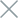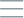• Home
• Tags
• Series
•# Linear Search Algorithm in PHP - Explanation, Example and Code

The Linear Search algorithm is a basic and straightforward search method. It works by iterating through each element of a sequence to find a specific value. While simple, this method is effective for small sequences or when the sequence is already sorted.

## How It Works

1. Iterate Through Elements: Start from the first element and check if the current value matches the target value.
2. Check for Match: If the value at the current position matches the target value, the search process ends, and the position of the value is returned.
3. Move to Next Element: If no match is found, move to the next element and continue checking.
4. Repeat: Repeat steps 2 and 3 until the value is found or the entire sequence is traversed.

## Example: Linear Search for the Number 7 in an Array

``````function linearSearch(\$arr, \$target) {
\$n = count(\$arr);
for (\$i = 0; \$i < \$n; \$i++) {
if (\$arr[\$i] == \$target) {
return \$i; // Return the position of the value
}
}
}

\$array = [2, 5, 8, 12, 15, 7, 20];
\$targetValue = 7;

\$result = linearSearch(\$array, \$targetValue);

if (\$result != -1) {
echo "Value \$targetValue found at position \$result.";
} else {